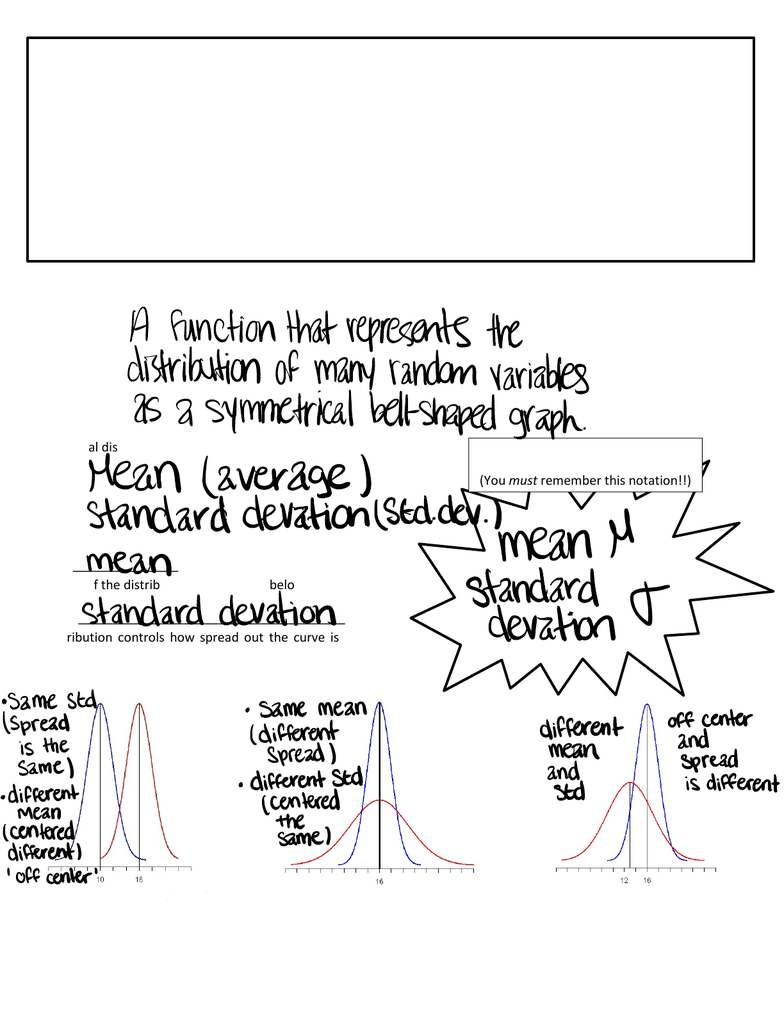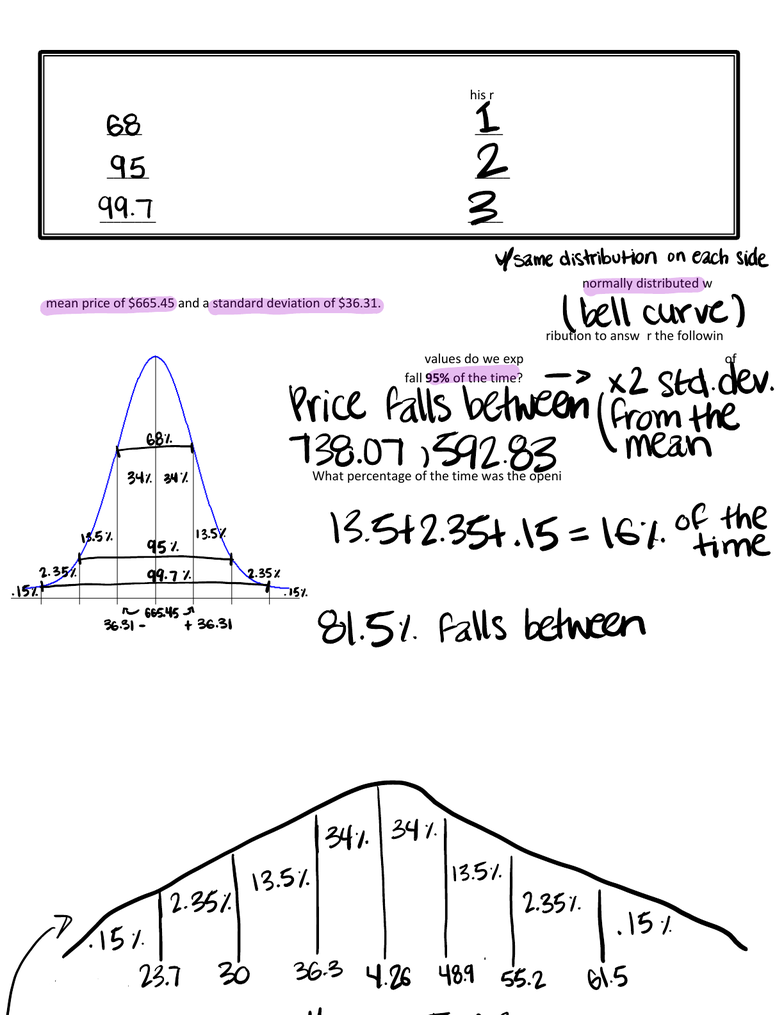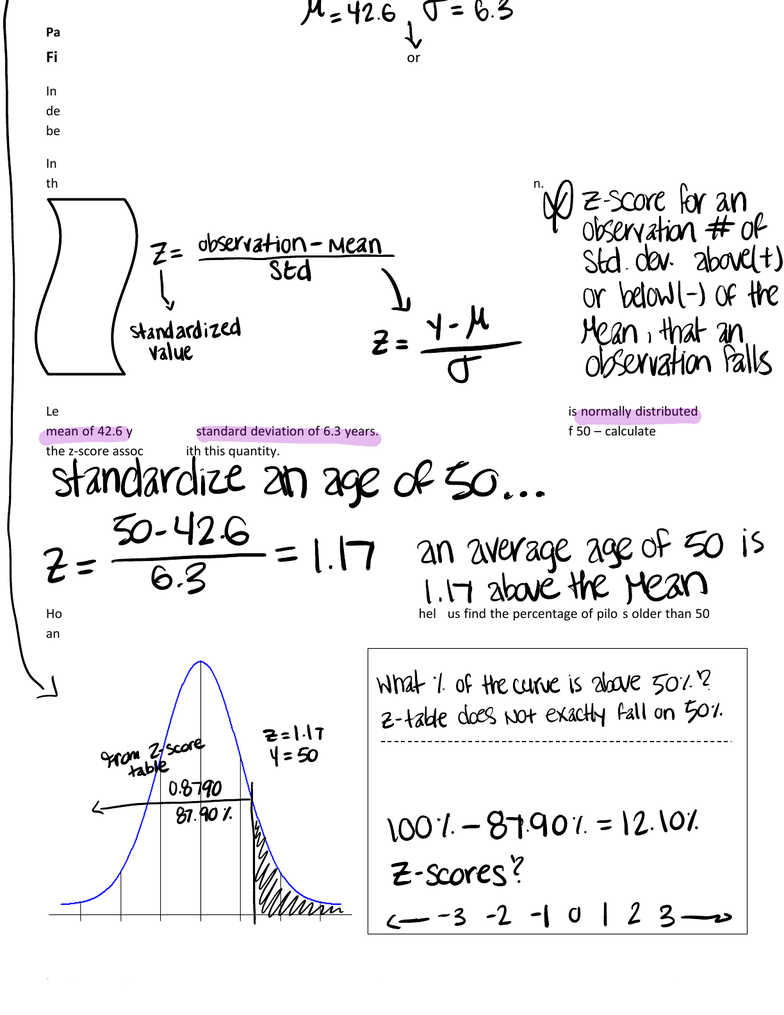Class Notes (1,100,000)
US (470,000)
NKU (40)
STA (10)
STA 205 (10)
Lecture 1

# STA 205 Lecture 1: Packet 1-Normal DistributionsExam

Department
Statistics
Course Code
STA 205
Professor
Brooke Buckley
Lecture
1

This preview shows pages 1-2. to view the full 8 pages of the document.Packet 1: Normal Distributions
After completing this material, you should be able to:
describe what a normal distribution is.
use the Empirical rule to describe normal distributions.
calculate a z-score and interpret its meaning.
find probabilities ( or percentages) of normal curves above a given observation, below a given observation, and
between two observations.
determine the value of a normal distribution corresponding to any given percentage (or probability).
determine when observations are unusual based upon probabilities and explain the reasoning.
What exactly is a normal distribution? In the space below, briefly define a normal distribution and draw several normal
curves.
A normal distribution is controlled by two features:
1.
2.
The ___________________ of the distribution controls the
center of the distribution (see Figure 1 below).
The ______________________________________ of the
distribution controls how spread out the curve is, which in
turn controls its scale (see the Figure 2 below).
(You must remember this notation!!)
Figure 1.
Blue Curve:
Red Curve:
Figure 2.
Blue Curve:
Red Curve:
Figure 3.
Blue Curve:
Red Curve:
!
!
!
!
!
!
!
!
!
!
!
!
!
!
!
!
!
!
!
!
!
!
!
!
!
!
!
!
!
!
!
!
!
!
!
!
!
!
!
!
!
!
!
!
!
!
!
Afunctionthatrepresents the
distribution of many random variables
asymmetrical bellshaped graph
Mean average
standard devationlstd dev.lme.am µ
mean standard
standard elevation derationt
ame Std same mean
Fsrethde different daimfnfeqrnent offngenter
ifferent diffetneffted Std is differen
cMeneimered theme
ifferent
oeecenter
difrerf.BR

Only pages 1-2 are available for preview. Some parts have been intentionally blurred.Packet 1: Normal Distributions page 2
STA 205 Notes Buckley Spring 2017
Example: Last year, the opening price for one share of Google stock has been approximately normally distributed with a
mean price of \$665.45 and a standard deviation of \$36.31.
Draw and label the normal distribution corresponding to opening prices. Use the distribution to answer the following:
Example: Delta reports that the average age of their pilots is 42.6 years with a standard deviation of 6.3 years. Assume
that the distribution of ages is normally distributed.
Draw and label the normal model which describes the ages of Delta pilots. What percentage of Delta pilots is older than
50? Why can we not use the Empirical Rule to arrive at an exact answer to this question? What is the best we can say?
To help understand how normal distributions work, we can use the
Empirical Rule
(which is also referred to as the
68-95-99.7 Rule – I’ll let you figure out where this name comes from!). This rule states the following:
________ % of the values in a normal distribution fall within __________ standard deviation of the mean.
________ % of the values in a normal distribution fall within __________ standard deviations of the mean.
________ % of the values in a normal distribution fall within __________ standard deviations of the mean.
Between what two values do we expect the opening price of one share of
Google stock to fall 95% of the time?
What percentage of the time was the opening price more than \$701.76?
What percentage of the time is an opening price between \$629.14 and
\$738.07?
68 I
95 2
997 3
Hsamedistribution oneachside
belt curve
xzstd.de
Price falls between from the
938.07559283 mean
34 341
ias 13.512.351.15 16 coffitmhee
is s
rs
so.si 545E3osi 81.51 falls between
1341
titsi
Iiii

Unlock to view full version

Only pages 1-2 are available for preview. Some parts have been intentionally blurred.Packet 1: Normal Distributions page 3
STA 205 Notes Buckley Spring 2017
Finding Normal Probabilities (also known as “working forwards”)
In the Delta pilot example, we ran into a problem the age we were interested in wasn’t exactly 1, 2, or 3 standard
deviations from the mean. This meant the Empirical Rule could no longer be used to find the percentage of the curve
below the observation. We need a way to find probabilities (or percentages) associated with any observation.
In order to find probabilities, we first need a way to determine the exact number of standard deviations above or below
the mean an observation falls. This process is known as standardizing the observation.
Let’s return to the pilot example we looked at last class meeting. The age of Delta pilots is normally distributed with a
mean of 42.6 years and a standard deviation of 6.3 years. We were interested in an age of 50 calculate and interpret
the z-score associated with this quantity.
How will standardizing the observation by calculating its z-score help us find the percentage of pilots older than 50? To
answer that question, let’s fill in the normal model below:
This formula will be given on the
formula sheet.
What information would we like to know?
Using the normal table, what percentage of the curve is below
an age of 50?
M42.6 F6.3
t
DzScore for an
observation OR
i
Standardize 2N age of 50
50 42.6
26.3 1.17 an average age of 50 is
1it abovethe Mean
What 1ofthecurve is above 5012
gragabfescore ZyEfolt
Ztabledoes Not exactly fallon 50
0.8790
901 loot 87.901 12 101
fqgynmZ sfzre.SIyoi23c
oaoBer o
o